# 2020 HSC Maths Standard 2 Exam Paper Solutions

In this post, the Matrix Maths Team present the solutions to the 2020 Maths Standard 2 paper.The Matrix 2020 HSC Maths Standard 2 Exam Paper Solutions are here!

## 2020 HSC Maths Standard 2 Exam Paper Solutions

Have you seen the 2020 HSC Mathematics Standard 2 exam Paper yet?

In this post, we will work our way through the 2020 HSC Maths Standard 2 exam paper and give you the solutions, written by our Head of Mathematics Oak Ukrit and his team.

## Want to see how the Matrix Academic Head of Maths would solve the common questions?

In this video, Head of Maths Oak Ukrit solves the common questions from the 2020 HSC Maths Advanced and Maths Standard 2 papers.

Read on to see how to answer all of the 2020 questions.

## Section 1. Multiple Choice

 Question Number Answer Solution 1. C A parabola is defined by the $$x^2$$ term in its equation. 2. D Zeroes between two non-zero numbers (in this case 2 and 7) are significant. 3. C $$S = \frac{D}{T} \rightarrow T = \frac{D}{T} \rightarrow D = \frac{75}{50} = 1.5 \ hours$$ 4. B $$200(1+\frac{0.03}{12})^{18} = 209.19$$ 5. B The range of solutions is $$15.95$$ to $$16.04 \dot{9}$$ Error $$\approx \frac{16.05-15.95}{16} = 0.00625 = 0.625%$$ 6. D Let $$x = 0, y = -1$$. Let $$x = 5, y = -11$$. The value of $$y$$ has decreased by $$10$$. 7. A 8. A French: $$\frac{82-70}{8} = 1.5$$, Commerce: $$\frac{80-65}{5} = 3$$, Music: $$\frac{74-50}{12} = 2$$ 9. B Each player plays against 3 others. The only network with 3 lines connecting to each point is B. 10. D Initial cost $$C = 90$$, hourly cost $$= 2 \times 60$$ minutes $$= 120$$. 11. C $$10000(0.92)^{10} = 4343.88$$ 12. D Coefficient is negative, so the graph must be going downwards. It cannot be B as there is a value not on the straight line, meaning that the coefficient would not be close to -1. 13. D Time = $$\frac{0.08375}{0.015} = 5.5833 = 5h \ 35 \ \text{min}. 10:30\ \text{pm} + 5h \ 35\ \text{min} = 4:05\ \text{am}$$. 14. A 15. B The possible combinations of (dice value, table value) are (1, 6), (2, 3), (3, 2), (6, 1). Each of these has a probability of $$\frac{1}{6}*\frac{1}{8} = \frac{1}{48}. 4*\frac{1}{48} = \frac{1}{12}$$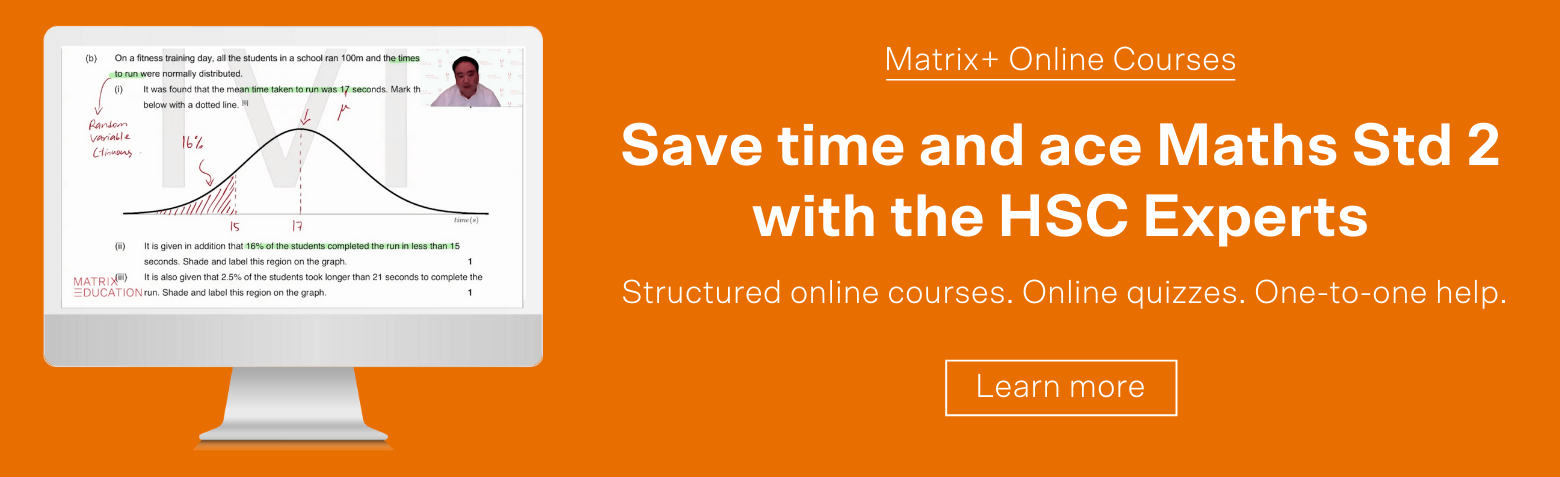## Section 2 Long Response Questions

### Question 16a

 \begin{align*} tan(\theta) = \frac{\text{opposite}}{\text{adjacent}} = \frac{8}{10} = 0.8 \\ \theta = tan^{-1}(0.8) \approx 39°\\ \end{align*}

### Question 16b

 \begin{align*} x^2 = \sqrt{8^2 + 10^2} \\ x^2 = 164 \\ x = 12.8 \end{align*}

### Question 17

Area of the $$5 \times 5$$ section is $$5 \times 5 = 25m^2$$, meaning there are $$8$$ trees for every $$25m^2$$ of land.

The area of the whole block of land is $$1000\times1000 = 1000000m^2$$

The total number of tress is $$\frac{1000000}{25}\times 8 = 320000$$ trees.

### Question 18a

Starting from vertex A, there are multiple spanning trees possible.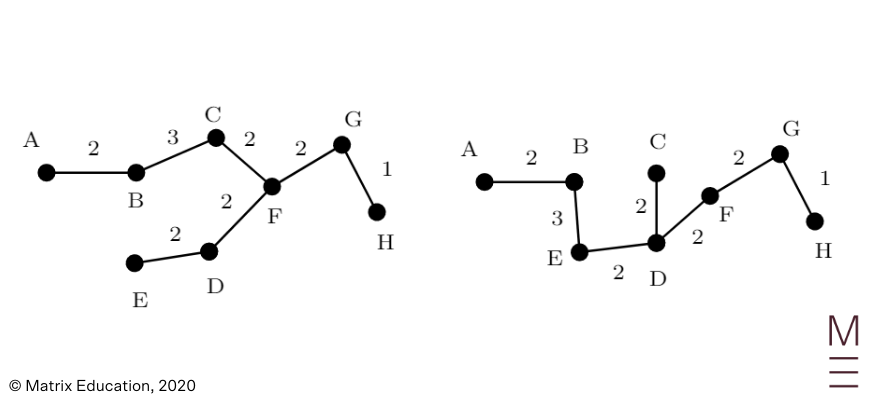Minimum length of spanning tree = 14.

### Question 18b

Adding point $$K$$ means adding another vertex. This can happen at either $$AK(12)$$ or $$CK(10)$$. For the minimum we want $$CK$$, so the minimum length is $$14 + 10 = 24$$ units.

### Question 19a

Reading from the graph, $$x = 200m$$.

### Question 19b

The graph is at the largest value of $$A$$ when $$x = 150m$$.

The value of $$y$$ can then be found from the fence equation by substituting $$x = 150$$.

 $$3x + 2y = 900 \rightarrow 2y = 900 – 3x \rightarrow y = \frac{900 – 3*150}{2} = 225 \ m$$

### Question 19c

 $$A = 450x – 1.5x^2 = 450 \times 150 – 1.5 \times 150^2 = 33750m^2$$.

### Question 20

Wally’s income is $$122680$$, so they are in the 4th tax bracket.

The total amount of tax required is

 $$20797 + 0.37 (122680 \ – 90000) = 32888.6$$

The total tax paid is $$3000 \times 12 = 36000$$

Therefore the tax refund will be $$= 36000 \ – 32888.6 = 3111.40$$.

### Question 23a

The ratio is $$15:9:4$$, so:

 \begin{align*} \textrm{Pineapple: } 15 &\rightarrow 3 \textrm{ litres}\\ 1 &\rightarrow \frac{1}{5} \textrm{ litre}\\ \textrm{Orange: } 4 \textrm { parts} &\rightarrow ?\\ \textrm{Since } 1 \textrm{ part} &\rightarrow \frac{1}{5} \textrm{ litre}\\ \textrm{Multiply by 4 to obtain:}\\ 4 \textrm{ parts} &\rightarrow \frac{4}{5} \textrm{ litres}\\ \textrm{Orange juice } = 800ml\textrm{ or } 0.8L \end{align*}

### Question 23b

 \begin{align*} \textrm {Volume } = 35 \times 20 \times 40 &= 28000ml\\ &= 28L\\ \text{Ratio: }&15:9:4\\ \text{Total parts }&=15 + 9 + 4 = 28 \text{ parts}\\ ∴ 1 \text{ part} &= 1 \text{ litre}\\ \text{Mango Juice }&= 9L\\ \end{align*}

### Question 24a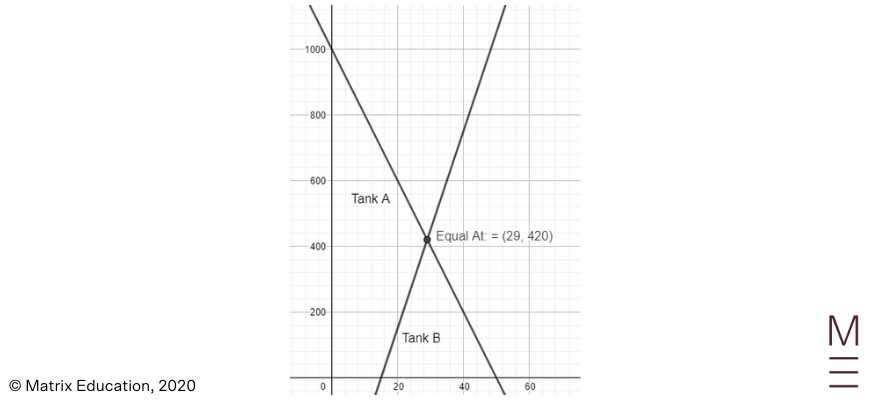### Question 24b

The equation for b is $$V_b = 30 (t-15)$$, reflecting the statement that it increases at a rate of $$30$$ after $$t=15$$ minutes.

The intersection above shows that the tanks contain the same volume of water at around $$30$$ minutes; this can be solved to obtain:

 \begin{align*} V_a &= 1000 – 2t\\ V_b &= 30 (t-15)\\ 100 – 20t &= 30(t-15)\\ 1000-20t &= 30t = 450 \\ 50t &= 1450\\ t&=29\\ V&=420 \end{align*}

### Question 24c

 \begin{align*} Total &= 1000 – 20t + 30 (t-15)\\ &= 1000 – 20t + 30t – 450\\ &= 550 + 10t\\ &= 1000 \text{ (from question)}\\ 10t &= 450\\ t&=45 \end{align*}

## Need personalised help answering these questions?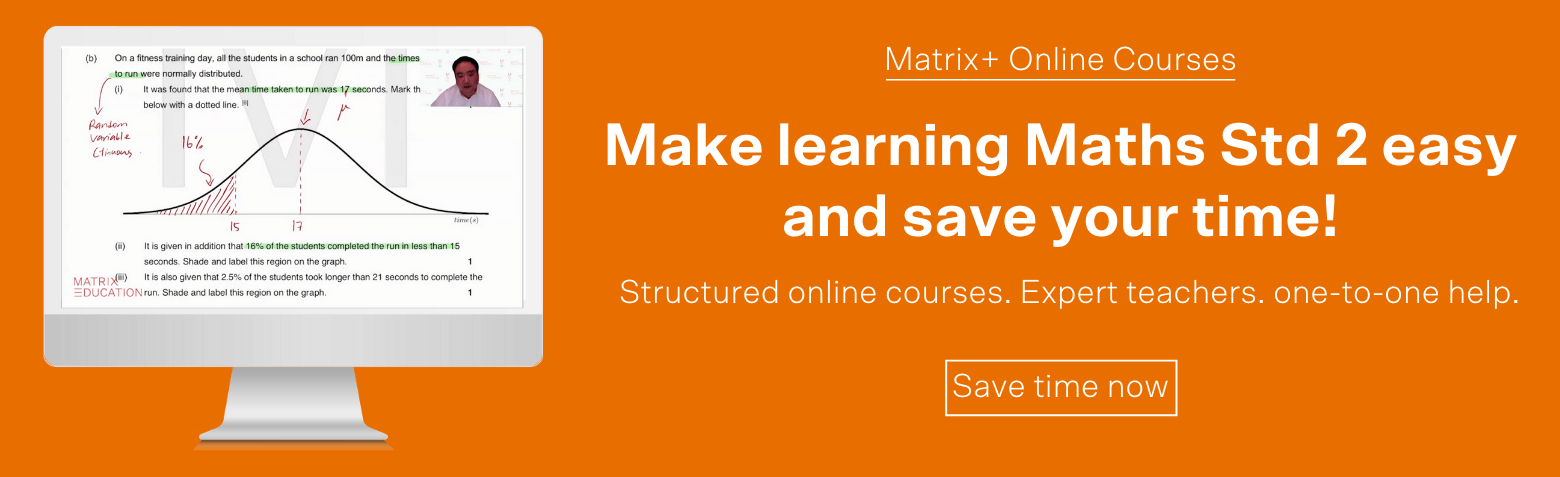### Question 25

The surface area of the solid is equal to the sum of 2 triangles, two roof rectangles, and 5 cube faces:

 \begin{align*} SA &= 2 \times \textrm{Triangle} + 2 \times \text{Roof rectangle} + 2 \times {Cube face}\\ &= 2 \times (\frac{1}{2} \times 8 \times 3) + 2 \times (5 \times 8) + 5 \times ( 8 \times 8)\\ &= 24 + 80 + 320\\ &= 424 cm^2\\ \end{align*}

Hence the surface area of the solid is $$424cm^2$$.

### Question 26a

Start by completing a forward and backwards scan to determine Earliest Starting Times (Red) and Latest Starting Times (Blue):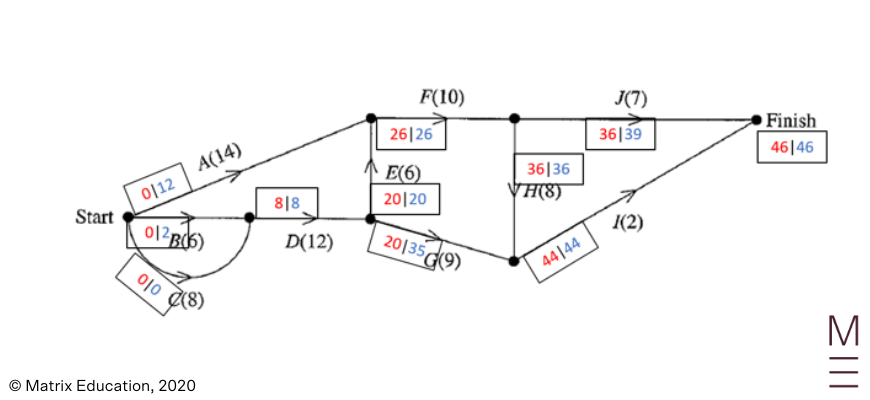The minimum time needed to prepare the meal is, therefore $$46$$ minutes, reading off the last node.

### Question 26b

The activities on the critical path are $$C\Rightarrow D \Rightarrow E \Rightarrow F \Rightarrow H \Rightarrow I$$, as they have no float time.

### Question 26c

We can calculate the float time by subtracting the earliest and latest start times for each activity.

 Activity Earliest Start Time Float Time (minutes) A 0 12 min G 20 15

### Question 27a

To do this, you need to draw two trapeziums on either side of the sharp point, take measurements of the heights of the trapeziums, and then calculate an area estimate: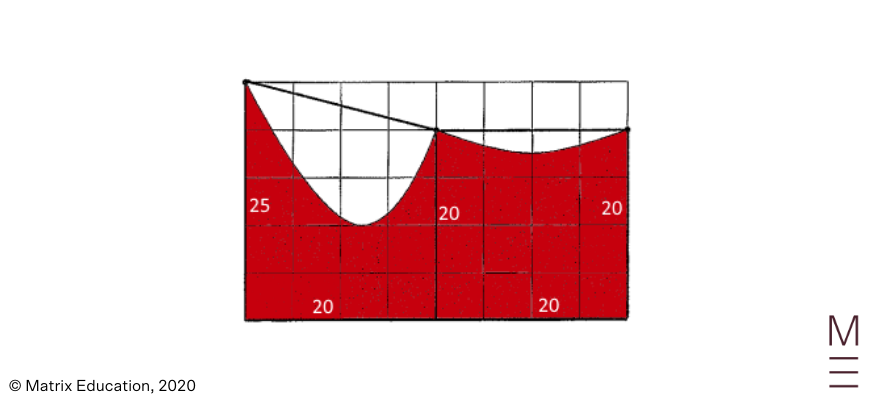### Question 27b

You can see that the trapeziums cover more area than the red shape; and hence the estimated area of part (a) is more than the actual area of the garden.

### Question 28

What we know:

 \begin{align*} x &> 15\\ mean_1 – mean_2 &= 10 \times (median_1 – median_2)\\ \end{align*} So, \begin{align*} mean_1 &= \frac{1+5+6+10+15}{5} = \frac{40}{5}=8\\ median_1 &= 9\\ median_2 &= 9.5\\ 8-mean_2 &= 10 (9-9.5)\\ 8-mean_2 &= -5\\ mean_2 &= 13 \\ ∴ 13 &= \frac{40+x}{6}\\ 78 &= 40 + x \\ x &= 38 \end{align*}

### Question 29

 \begin{align*} \textrm{ABC total share price }&= 200 \times \$5.50\\ &=$1100\\ \textrm{dividends from ABC } &= $1100 \times 0.06\\ &=$66\\ \end{align*} Let $$x$$ be the number of shares in $$\text{XYZ}$$. \begin{align*} \textrm{XYZ total share price }&= 6x\\ \textrm{dividends from XYZ} &= 6x \times 0.04\\ &=0.24x\\\\ \textrm{dividends from ABC }+ \textrm{dividends from XYZ} &= \textrm{total dividends}\\ 66 + 0.24x &= 149.52 \\ 0.24x &= 83.52\\ x &= 348\\ \end{align*}

Therefore, Jana has $$348$$ shares in $$XYZ$$.

### Question 30a

To find maximum flow, we trace all the possible paths from the source to the sink. Then, for each path, we determine the smallest pipe in the path, and fill the remaining pipes with this smallest value. We then add the pipe’s flow to the total flow. Using this method, we obtain a possible maximum flow rate (in brackets)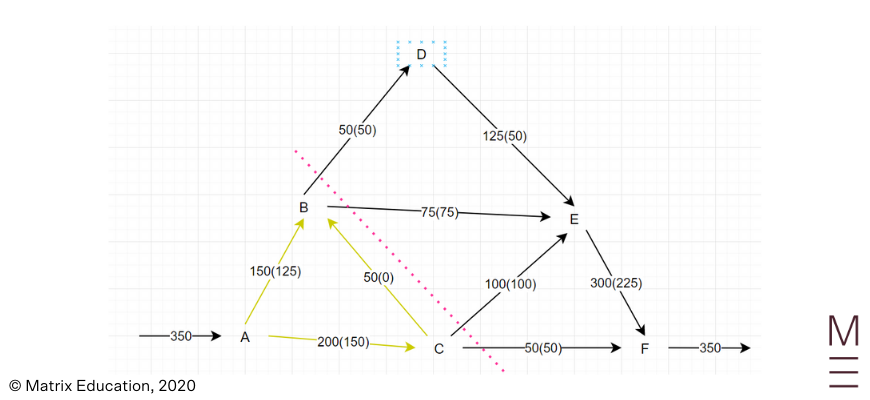From here, we can take a cut on a set of flows that are not indeterminate, to calculate the maximum flow rate. For this question, this is $$50 + 75 + 100 +50 = 275$$.

### Question 30b

The value of the cut is given as the sum of all pipes that flow from the source node set to the sink node set. This means we exclude flow CB, giving a cut value of $$50 + 75 + 200 = 325$$. This is more than the maximum flow rate of $$275$$, so this cut is not a minimum cut.

### Question 31a

We have

 \begin{align*} \angle APB &= \angle NPB – \angle NPA \\ &= 100° – 35° \\ &= 65° \\ \end{align*}

as required.

### Question 31b

Using the cosine rule, we have

 \begin{align*} AB^2 &= AP^2 + PB^2 – 2 \times AP \times PB \times \cos 65° \\ &= 7^2 + 9^2 – 2 \times 7 \times 9 \times \cos 65° \\ &= 76.75 \ \end{align*}

so

 \begin{align*} AB &= \sqrt{76.75} \\ &= 8.76 \, \text{km} \quad \text{(correct to 2 d.p.)} \,. \end{align*}

### Question 31c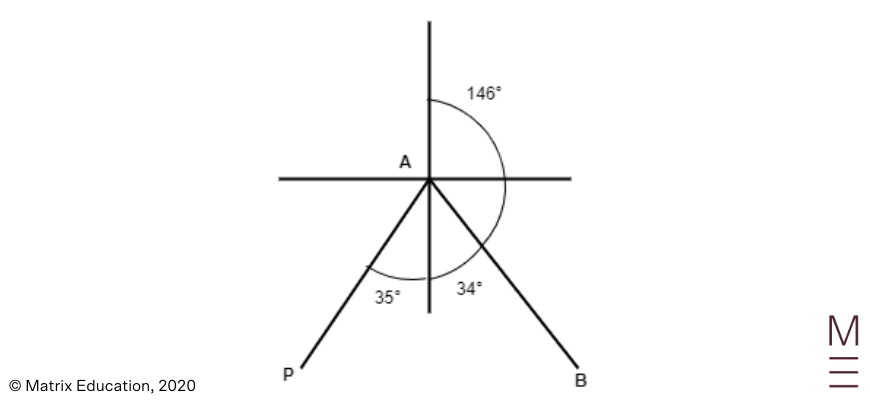Using the cosine rule, we have

 \begin{equation*} PB^2 = AP^2 + AB^2 – 2 \times AP \times AB \times \cos (\angle PAB) \\ \end{equation*}

Rearranging this gives

 \begin{align*} \cos (\angle PAB) &= \frac{AP^2 + AB^2 – PB^2}{2 \times AP \times AB} \\ &= \frac{7^2 + 76.75 – 9^2}{2 \times 7 \times 8.76} \\ &= 0.365 \\ \end{align*}

so

 \begin{align*} \angle PAB &= \cos^{-1} (0.365) \\ &= 68° 36′ \\ &\approx 69° \ \end{align*}

Therefore,

 \begin{align*} \text{bearing} &= 180° – (69° – 35°) \\ &= 146° \quad \text{(correct to the nearest degree)} \,. \end{align*}

### Question 32

Using the given perimeter we have

 \begin{align*} AB &= \frac{\text{perimeter}}{\text{number of sides}} \\ &= \frac{80}{10} \\ &= 8 \, \text{cm} \\ \end{align*}

We also have

 \begin{align*} \angle AOB &= 360° \div 10 \\ &= 36° \\ \end{align*}

Using the cosine rule gives

 \begin{align*} \angle AOB &= 360° \div 10 \\ &= 36° \,. \end{align*}

Since $$AO = BO$$ and $$AB = 8$$, we have

 \begin{align*} 8^2 &= AO^2 + AO^2 + 2 \times AO \times AO \times \cos 36° \\ 64 &= 2 (AO)^2 + 2 AO^2 \cos 36° \\ 64 &= AO^2 (2 + 2 \cos 36° ) \\ AO^2 &= \frac{64}{2 + 2 \cos 36°} \\ \end{align*}

Therefore,

 \begin{align*} \text{area of the decagon} &= 10 \times \text{area of \triangle AOB} \\ &= 10 \times [\frac{1}{2} \times AO^2 \sin 36°] \\ &= 10 \times \frac{1}{2} \times \frac{64}{2 + 2 \cos 36°} \times \sin 36° \\ &= 51.987 \\ &\approx 52.0 \, \text{cm}^2 \quad \text{(correct to 1 d.p.)} \\ \end{align*}

## Need support answering these questions?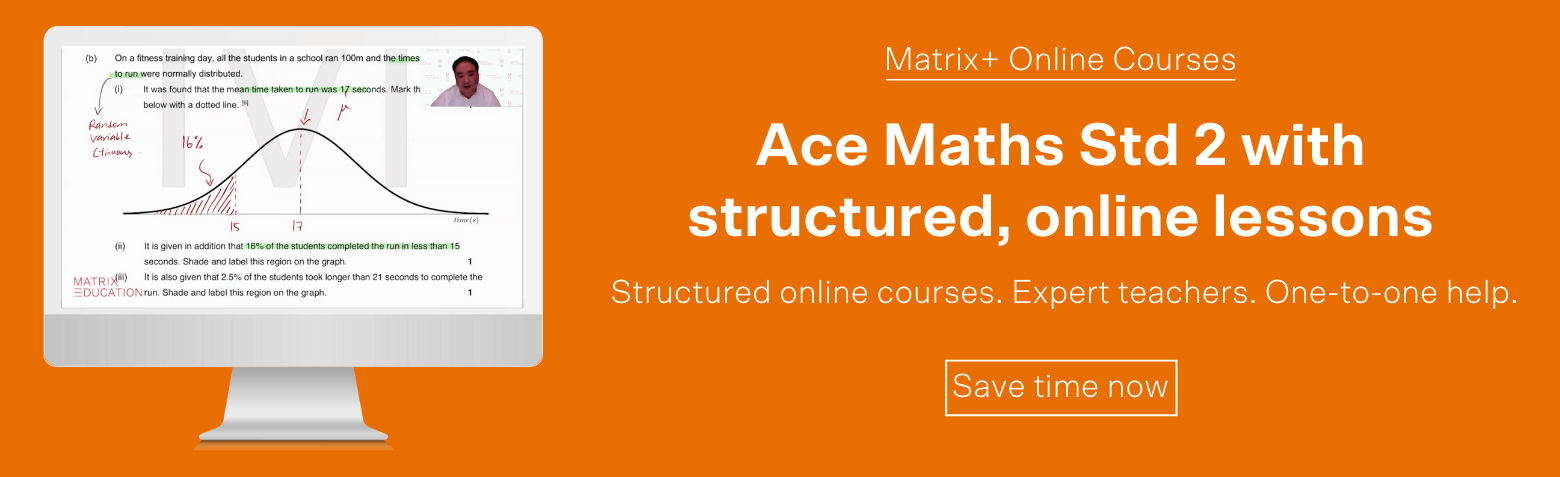### Question 33a

Reading from the graph, the solution is $$4000$$.

### Question 33b

 \begin{align*} y&=A\times b^n\\ \end{align*} At $$n=0$$, $$y=1000$$, so: \begin{align*} 1000 &= A\times b^0\\ &= A \times 1\\ &= A\\ ∴ A &= 1000\\ \end{align*}

From our answer in (a), we have $$4000 = 1000 \times b^{40}$$. Given even $$2^{40}$$ is a very large number, we should start our guess and check with a small number:

 Guess b $$y = 1000 \times b^4 0$$ Result 1.5 1010 Too large 1.1 45259 Too large 1.05 7040 Too large 1.04 4801 Too large 1.03 3262 Too small

The upper and lower estimates are therefore $$1.03$$ and $$1.04$$.

### Question 34a

We use the recurrence relation starting with

 \begin{equation*} A_0 = 60000 \\ \end{equation*}

The amount of money in the account after the first withdrawal is

 \begin{align*} A_1 &= A_0 (1.005) – 800 \\ &= 60000 (1.005) – 800 \\ \end{align*}

the amount of money in the account after the second withdrawal is

 \begin{align*} A_2 &= A_1 (1.005) – 800 \\ &= [60000 (1.005) – 800] (1.005) – 800 \\ &= 60000 (1.005)^2 – 800 (1 + 1.005) \\ \end{align*}

and the amount of money in the account after the third withdrawal is

 \begin{align*} A_3 &= A_2 (1.005) – 800 \\ &= [60000 (1.005)^2 – 800 (1 + 1.005)] (1.005) – 800 \\ &= 60000 (1.005)^3 – 800 (1 + 1.005 + 1.005^2) \\ &\approx 58492.49 \quad \text{(correct to 2 d.p.)} \\ \end{align*}

### Question 34b

The interest in the first month is

 \begin{align*} I_1 &= A_0 \times 0.5% \\ &= 60000 \times 0.005 \\ &= 300 \\ \end{align*}

dollars. The interest in the second month is

 \begin{align*} I_2 &= A_1 \times 0.5% \\ &= (60000 \times 1.005 – 800) \times 0.005 \\ &= 297.50 \\ \end{align*}

dollars. The interest in the third month is

 \begin{align*} I_3 &= A_2 \times 0.5% \\ &= (60000 \times 1.005^2 – 800 \times 1.005) \times 0.005 \\ &= 298.9875 \\ \end{align*} ### Question 35a Yin’s IQ is 128. City A’s mean IQ is $$\mu = 108$$, and they have standard deviation $$\Sigma = 10$$. The z-score of Yin’s IQ in City A is therefore:  \begin{align*} z &= \frac{128 – 100}{10}\\ &= 2 \end{align*} We know that $$95%$$ of a normal distribution has z-scores between $$-2$$ and $$2$$; so $$100-95 = 5 %$$ have z-scores beyond $$-2$$ and $$2$$. Now, since a normal distribution is symmetric, there should be an equal portion of people with z-scores less than $$-2$$ and greater than $$+2$$. ∴ The percentage of adults in city A with a higher IQ is $$5% \div 2 = 2.5%$$. ### Question 35b Yin’s IQ is 128. City B has $$\mu = 112$$, $$\sigma = 16$$. In a normal distribution. 68% have z-scores between -1 and 1; so $$100-68 = 32%$$ have z-scores beyond $$1$$ and $$-1$$. A normal distribution is symmetric, so there are an equal proportion of adults with z-scores less than $$-1$$ and greater than $$1$$. ∴ Percentage of adults with z-scores less than 1 is $$32% \div 2 = 16%$$ ∴ Percentage of adults with IQ less than Yin in City B is $$16% + 68% = 84%$$ ∴ Number of adults in City B with an IQ less than Yin’s is $$84% \times 1,000,000 = 840,000.$$ ### Question 35c Simon’s z-score in city A is $$z_a = \frac{x-108}{10}$$; in city B it is $$z_b = \frac{x – 112}{16}$$. If we equate $$z_a = z_b$$, then we have:  \begin{align*} \frac{x – 108}{10} &= \frac{x-112}{16}\\ 16 (x – 108) &= 10 (x-112)\\ 16x – 1728 &= 10x – 1120\\ 16x – 10x &= -1120 + 1728\\ 6x &= 608\\ x \approx 101.3 \textrm{ (3 d.p.)}\\ \end{align*} ### Question 36 From the box plot, the median of the temperature is $$22$$ degrees Celsius. It is given that the mean temperature is $$0.525$$ degrees below the median temperature, so  \begin{align*} \bar{x} &= 22 – 0.525 \\ &= 21.475 \\ \end{align*} degrees Celsius. The average number of chirps over the 20 days is  \begin{align*} \bar{y} &= \frac{684}{20} \\ &= 34.2 \\ \end{align*} Taking the expected value of both sides of  \begin{equation*} y = -10.6063 + bx \\ \end{equation*} gives  \begin{equation*} \bar{y} = -10.6063 + b \bar{x} \\ \end{equation*} so  \begin{equation*} 34.2 = -10.6063 + b (21.475) \\ \end{equation*} and  \begin{align*} b &= \frac{34.2 + 10.6063}{21.475} \\ &= 2.08644004657 \\ \end{align*} This means that  \begin{equation*} y = -10.6063 + 2.08644004657x \\ \end{equation*} Therefore, the number of chirps expected in a 15-second interval when the temperature is 19 degrees Celsius is found by substituting $$x = 19$$, giving  \begin{align*} y &= -10.6063 + 2.08644004657 (19) \\ &\approx 29 \quad \text{(correct to the nearest whole number)} \\ \end{align*} ### Question 37 The minimum lump sum is the amount that results in no money in Wilma’s account after $$30$$ years of withdrawals. For $$1000$$ at the end of each year for $$20$$ years:  \begin{align*} A_1 &= 1000 \times 16.351\\ &= 16351 \\ \end{align*}

For $$3000$$ each year for ten years starting $$21$$ years afte rthe account is opened:

 \begin{align*} A_2 = \frac{3000\times 8.983}{1.02^{20}} = 18135.90 \\ \end{align*} We divide by $$1.02^{20}$$ because of accruing $$2%$$ p.a. interest over $$20$$ years.  \begin{align*} \textrm{Minimum lump sum }&= A_1 + A_2\\ &= 16351 + 18135.90\\ &= \34486.90\\ \end{align*}

## Want to know your ATAR?

Use the calculator to explore the HSC Marks you need to achieve your ATAR Goal.